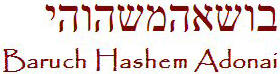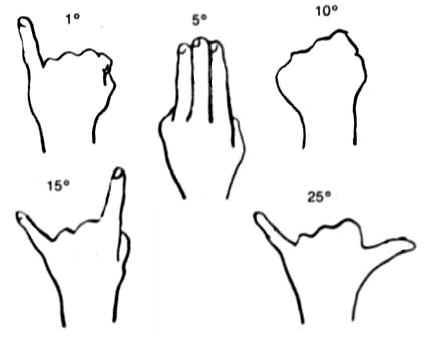The New Moon. When is the conjunction? Reading the sky. How to determine the conjunction if you only have the sky to watch. The new moon, which determines the beginning of the month, is covered or concealed until it is revealed. The crescent moon originated in Babylon and became an integral part of Islam. Psalm 83:1 Blow up the trumpet in the new moon, in the time appointed, on our solemn feast day.    תּקע tâqa‛ שׁפר shôphâr חדשׁ chôdesh כּסא keseh חג châg יום yôm כּ (open) hand/ cover  ס prop ה [comes from] Finding an Angle • Astronomers measure angular separation of objects in degrees. There are 360 degrees in a circle. And the angular separation of any point on the horizon and the point directly overhead (the zenith) is 90 degrees. Halfway from the zenith to the horizon is 45 degrees. • When you hold your hand at arm’s length, you can estimate angles like this: - Stretch your thumb and little finger as far from each other as you can. The span from tip to tip is about 25 degrees - Do the same with your index finger and little finger. The span is 15 degrees - Clench your fist at arms length, and hold it with the back of your hand facing you. The width is 10 degrees - Hold your three middle fingers together; they span about 5 degrees - The width of your little finger at arms length is 1 degree.Calculating the Conjunction The conjunction happens at the same moment in time no matter where a person is on the earth. According to Josephus, Seth’s sons were the first astronomers and had developed a knowledge of the precise cycles of the sun, moon and stars (what we today call astronomy). We can likewise determine the various cycles of the moon with precision. 1. Toward the end of the week before the conjunction, shortly before sunrise sight the waning moon above and towards the eastern sky. 2. With your hand measure the angle between the waning moon and the horizon, just as the sun rises; watching for a time that most closely fits measuring degrees with your hands. 3. Mark down the date, the degree of the angle (eg.25°) and the time (eg.4:30 a.m.) The angle in this example is 25° between the moon and the horizon where the sun is just appearing; and this is about 2 days before the conjunction (or what you believe will be the day of the conjunction). Let's use 4:30 a.m. when the sun appears. The moon travels around the earth once a month in about 29.53 days, on average (1 revolution is 360°). We do a calculation: 29.53 days X 24 hours a day = 708.72 hours (on the average) in one 360° revolution of the moon in one month. Then divide 360° by 708.72 hours to determine that the moon moves precisely .508° or about .51° in one hour. The moon moves relative to the sun by an amount .51°; multiplying by 24 hours, makes the movement of the moon about 12.2° every day. The formula is the angle of the sun to the moon at sunrise divided by the rate of change per hour, which equals the time of conjunction, in hours, from the time you took your measurements. That's all you need to know. The moon will travel 360° from new moon to new moon in 29.53 days, on average. Take the 25° measurement for the angle between the sun and the moon at sunrise and divide it by the .51° rate of change per hour. This gives us the amount of time it will take for the sun and moon to reach the point of alignment or conjunction.Therefore 25° divided by .51 degrees per hour gives us 49.2 hours. It will take 49.2 hours from 4:30 a.m., when we determined our angle between the sun and the moon, for the moon to travel the 25° still needed to reach conjunction. So, how many days away is 49.2 hours from the time we determined our angle between the sun and the moon? How many days away is the conjunction? Divide 49.2 hours by 24 hours (don't use fractions of a day for the remainder; we want whole days with the remainder in hours) and we come up with 2 days, with 1.2 hours or 72 minutes remaining. Add this 2 days to the date marked down, and we arrive at 4:30 a.m (2 days later). Add the remaining 1 hour and 12 minutes to 4:30 a.m., and that brings us to 5:42 a.m. as our “rough” calculation for the time of the conjunction. The new month then begins after sunset the following evening in this example.